## BJ & Co's No Solution Walk-through Guide to The  Witness

This is a current work in progress! Thanks for taking the time to stop by and take a peak. We appreciate any feedback you might have! Feel free to drop it in the comments of our reddit post or email it to our email! The Good, the Bad, and the Ugly we want to hear it all as we want the best possible guide for the community!

The Witness is a beautiful single-player puzzle game with dozens of locations to explore and over 500 puzzles. This game does a wonderful job of guiding you through the game from place to place, slowly introducing new ideas as you progress. As wonderfully as that works, it probably won't get you to 100% in the game. Therefore we here at BJ & Co have put together this walk through guide to show you the locations of all of the puzzles in this game so that you too can 100% The Witness!

The idea with this guide is to show you all the puzzle locations and provide you with the the ideas, logic, tips and tricks behind solving each of the puzzles in The Witness. There are no solutions on this page so you can avoid solutions spoilers however we will link to each of the solutions for those of you that get completely stumped. There will be a video for each section of the world as well as text and picture based description of each puzzle, its locations and a description of the process to solve but not the actual solution. We will go through all of the puzzle panels first, then go through the obelisks and associated environmental puzzles. Currently there is a great environmental guide in the works over at http://eguide.profdave.com/

You can see how well you are doing by looking at your save file. This will tell you how many puzzles and environmental puzzles you have solved. it will be a format of (XXX, +YYY, +Z) where XXX is the number of actual puzzle panels you have solved, YYY is the number of environmental puzzles you have completed and Z is the number of obelisks you have completed. a 100% completion has your save file at 523, +135, +6.

Before we dive in, We would like to thank all of our wonderful viewers who joined us for our own adventure through The Witness. If you would like to watch our initial struggles you can check it out on our playlist. We would also like to say thanks to the r/witness community for being so fabulous in supporting us!

Let's get on going with our  no solution guide to The Witness

1. Staring/Entry Area

2. Orchard

3. Lake

4. Symmetry

5. Desert

6. Quarry

8. Castle/Keep

9. Shipwreck

10. Tree-house/Colored Pointy Circles

11. The Bay

12. Marsh

13. River

14. Monastery

15. Peninsula

16. Tropical Forest

17. Town/Hub

18. Mountain

###### 1. Starting/Entry Area - XXX Puzzles

The Starting Area is a nice introduction to a very simple concept. This concept is starting from the circle and finishing at a point that juts out of the puzzle with a rounded end.## Puzzle 1

### The first puzzle introduces the basic concept for the game which is where to start and where to finish. Should be easy to pick up on since there is only two options for each.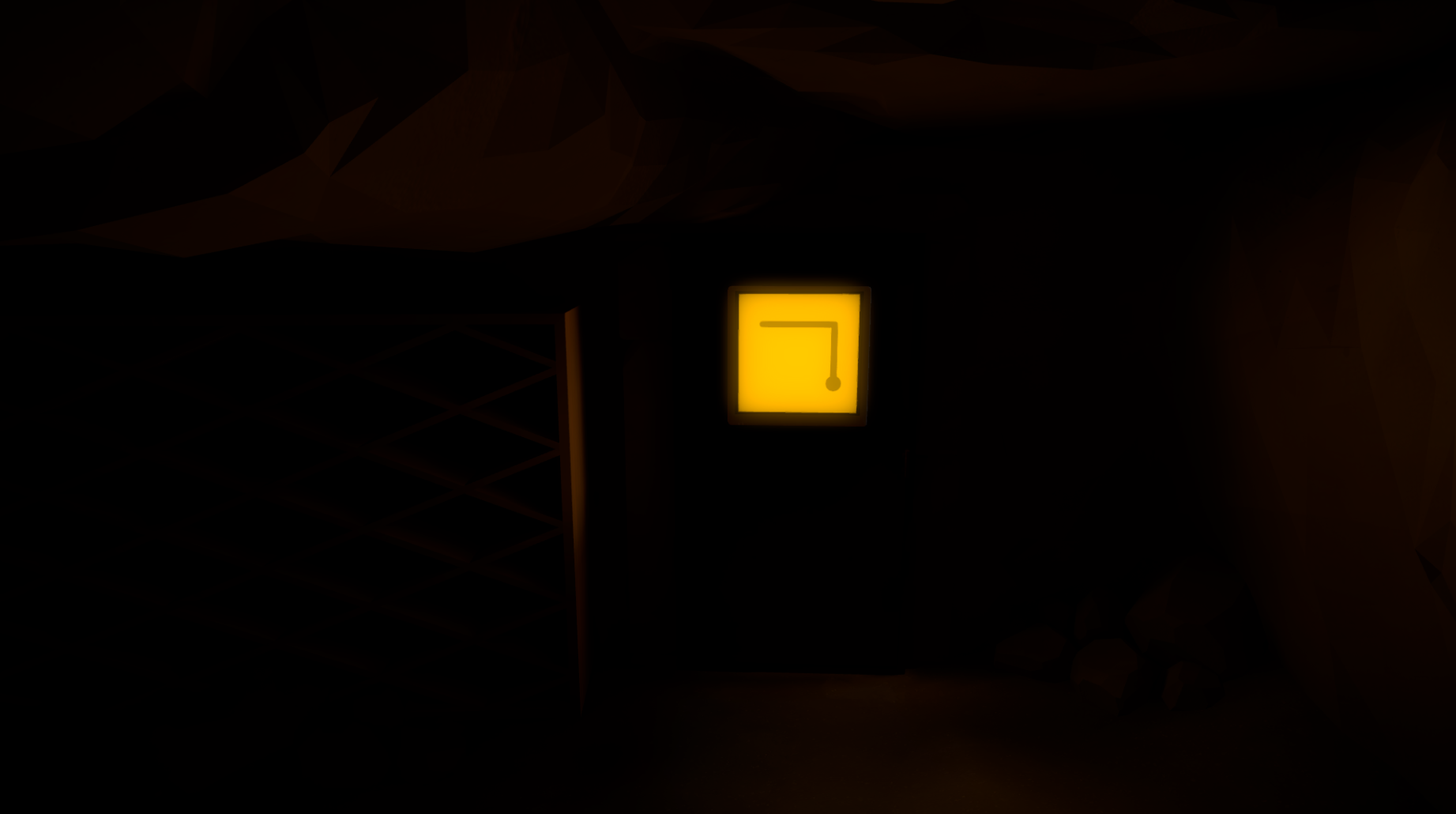## Puzzle 2

### Similar to the previous puzzle all you need is to get from start to finish. nothing new introduced besides the fact that your line can make corners. Should be a quick solve if not we suggest keeping a guide handy ;)## Puzzle 3

### A slightly more complex puzzle but still only needing to get from start to finish. Depending how you attempt to solve you may learn from this puzzle that lines cannot cross. Completing the puzzle will introduce a new concept regarding how to find where the next puzzles to complete are. The wires are key.## Puzzle 4

### Hopefully you were nicely guided to this panel. the puzzle is straightforward more complexity added but no change of concept.## Puzzle 5

### And more complexity but no change of concept. once complete will guide you to what is blocking your progress forward.## Puzzle 6

### After finding your way blocked you should be guided to this puzzle or Puzzle 7. This puzzle introduces multiple starting points including that fact that some starting points are positioned in ways that make it impossible to solve the puzzle if you start from that particular starting point.## Puzzle 7

### After finding your way blocked you should be guided to this puzzle or Puzzle 6. This puzzle introduces the mechanic of multiple end points into the mix. Note the wires coming from each end point. Both solutions are necessary to 100% the game however one is much more important in order to get out of the current area.## Puzzle 8

### Now that you have completed the required prior puzzles (5,6, and 7) you are able to complete this puzzle. Note that it can be solved in multiple ways all of which are correct and break the force field as long as you can make it from start to finish without crossing. once the force field is down, follow the path in order to get to the next sets of puzzles### As you travel down the path from Puzzle 8 you will likely encounter this puzzle along the way. Take a look but don't get too hung up on trying to solve it. You have not been "taught' by the game how to solve this yet so remember where it is and come back when you are better prepared.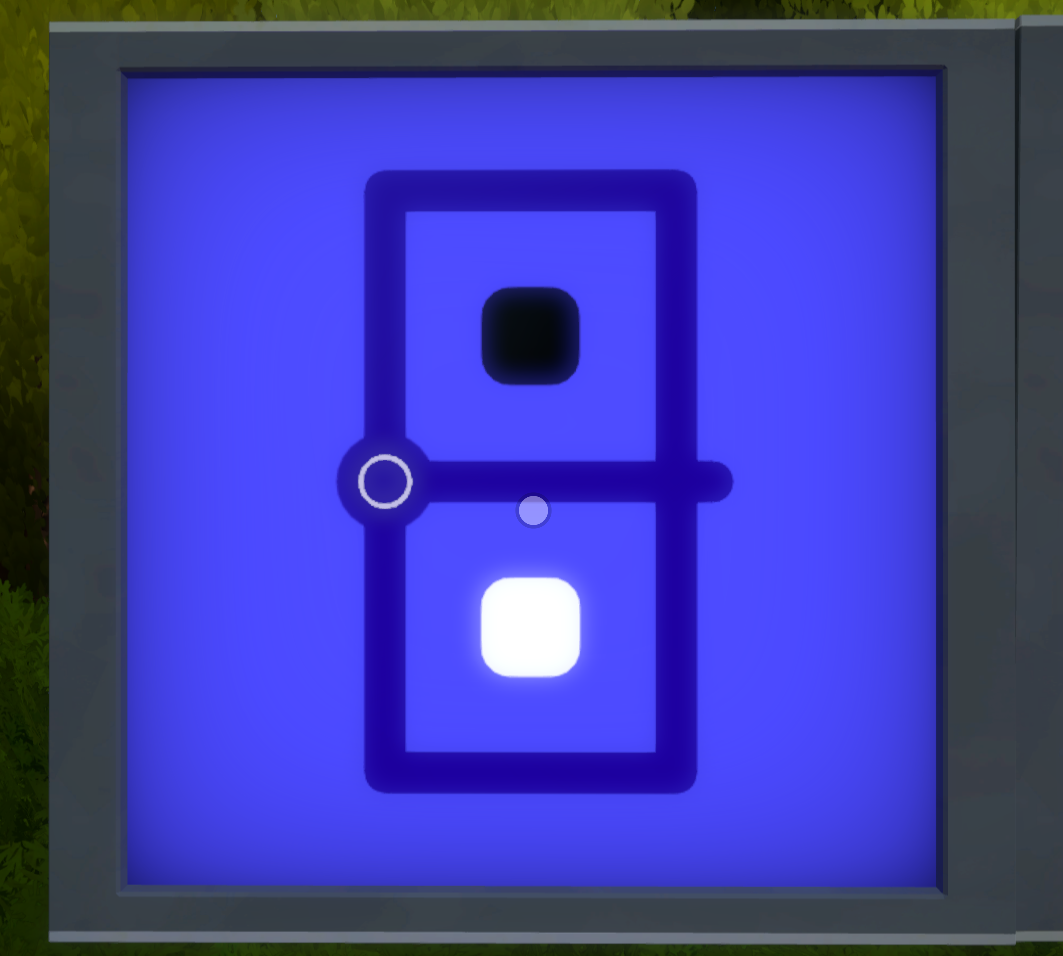## Puzzle 9

### As you travel further down the path you will come across 2 sets of puzzle panels we will take a look at the blue set first. This will introduce you to the logic behind colored squares with rounded edges. With only 3 possible ways to finish this first puzzle, it should be easy to solve and come to the conclusion that the different colors need to be kept separate from each other.## Puzzle 10

### Using the logic regarding separation this next puzzle should also be a quick solve for you. If you ever start to have issues with this sort of puzzle, mark out which line segments that will have to be filled in to complete it. These are the segments in which there is a colored square directly on both sides of the segment.## Puzzle 11

### Another quick solve for you as there isn't many options available to separate the colors.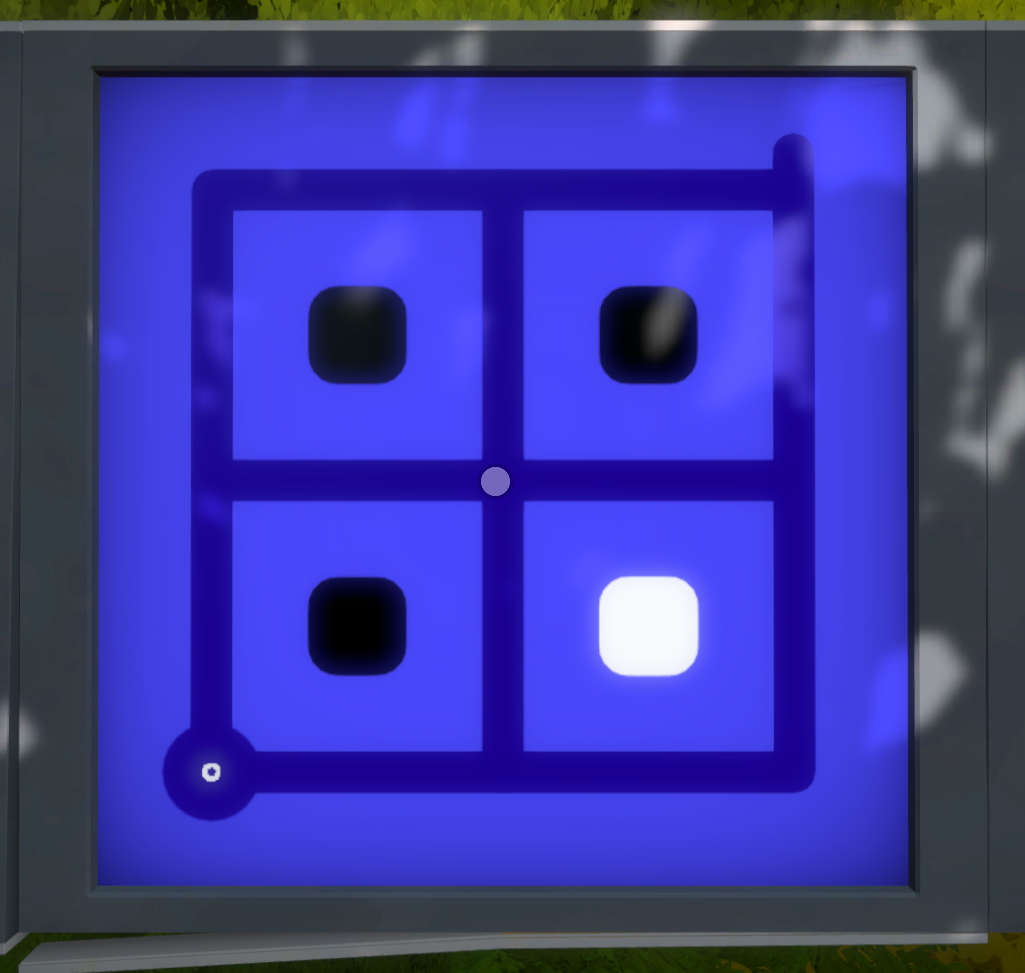## Puzzle 12

### Things start to get a bit more complex as blocks start to have colors on multiple sides in this puzzle but the logic doesn't change.## Puzzle 13

### Adding in a bit more complexity but the important lines to draw should be pretty apparent.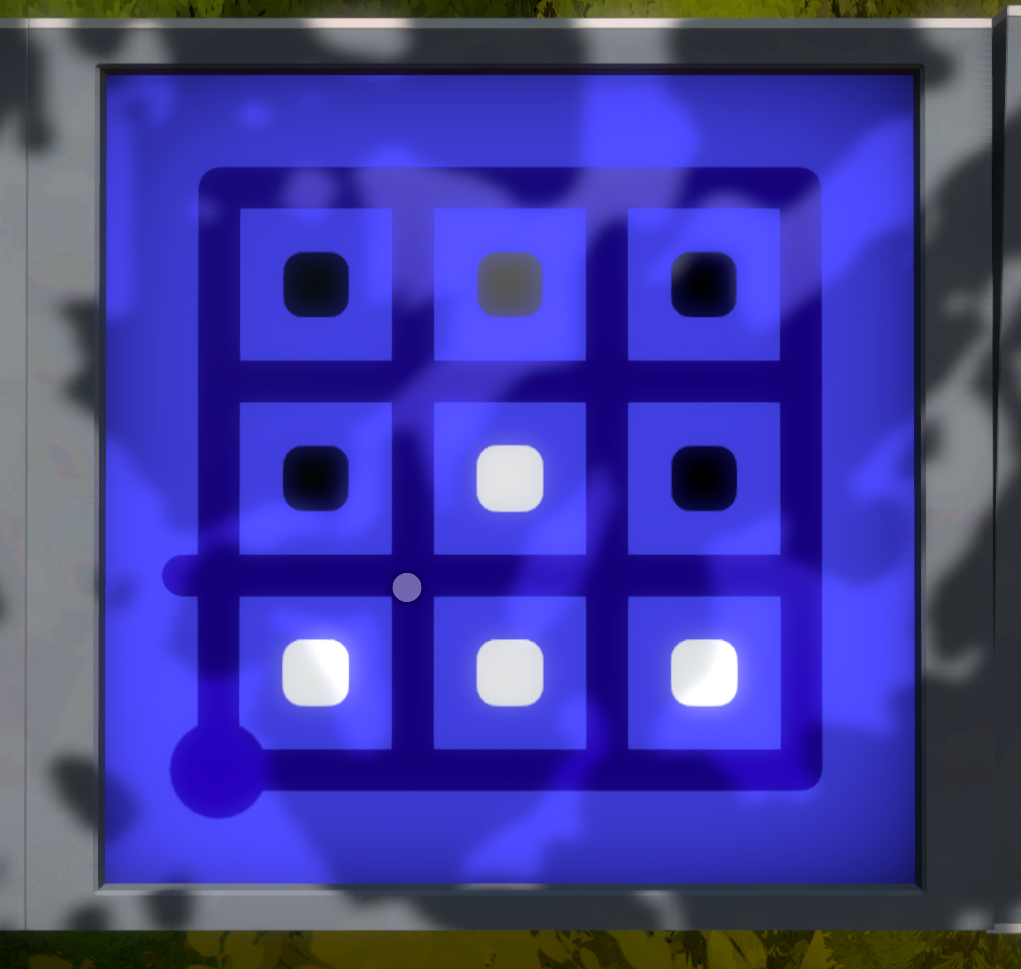## Puzzle 14

### Basically the same as the previous puzzle so the 5 important line segments should be pretty obvious but with the change in end point you will likely need a different route to get to the end. look at which line segments need to be filled in then possibly work backwards from finish to start to get to the solution.## Puzzle 15

### Bigger change now with the whites being separated by black. This shows you that the colors do not need to all be in the same section as long as different colors are separated. ie you can have 1 section of black and 2 sections of white.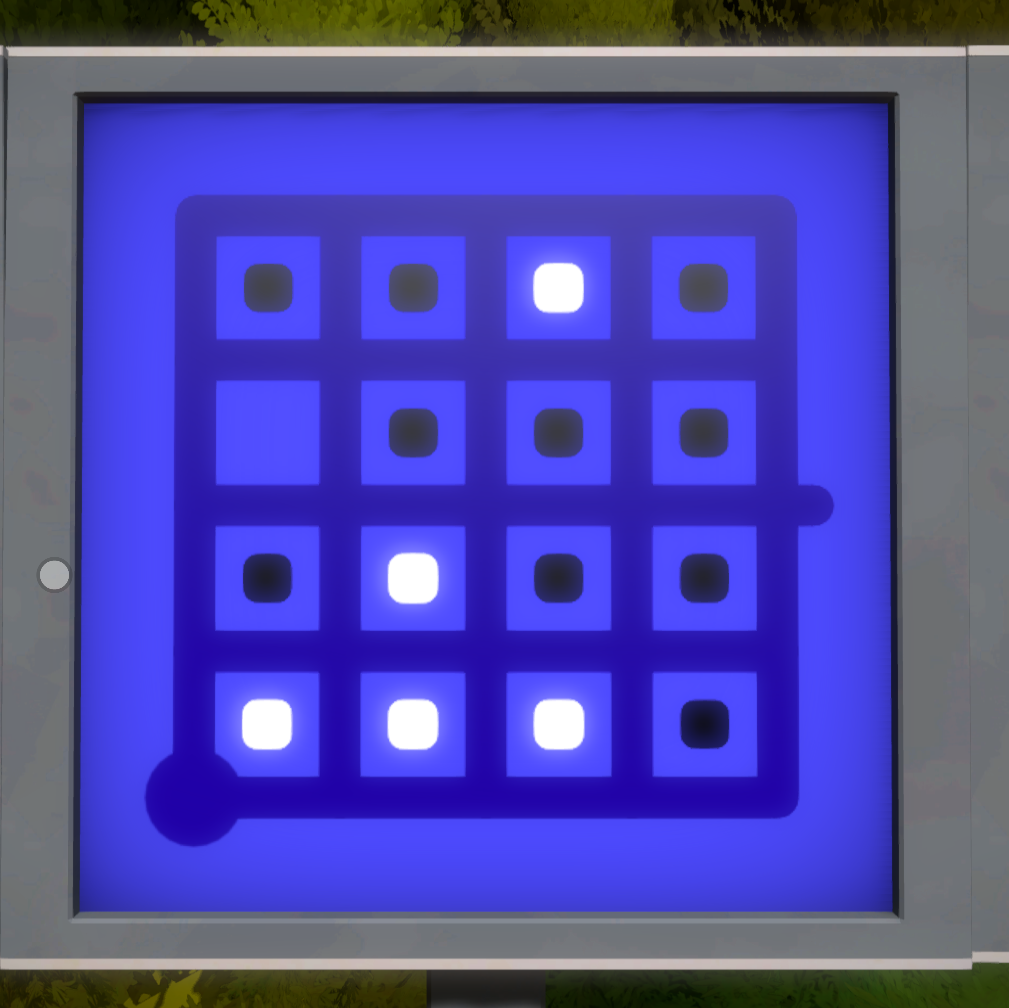## Puzzle 16

### Similar to the previous puzzle but the end point has moved on you increasing the difficulty. If you are getting stumped, look back at what you had to do in puzzles 13 and 14 as that might help you out.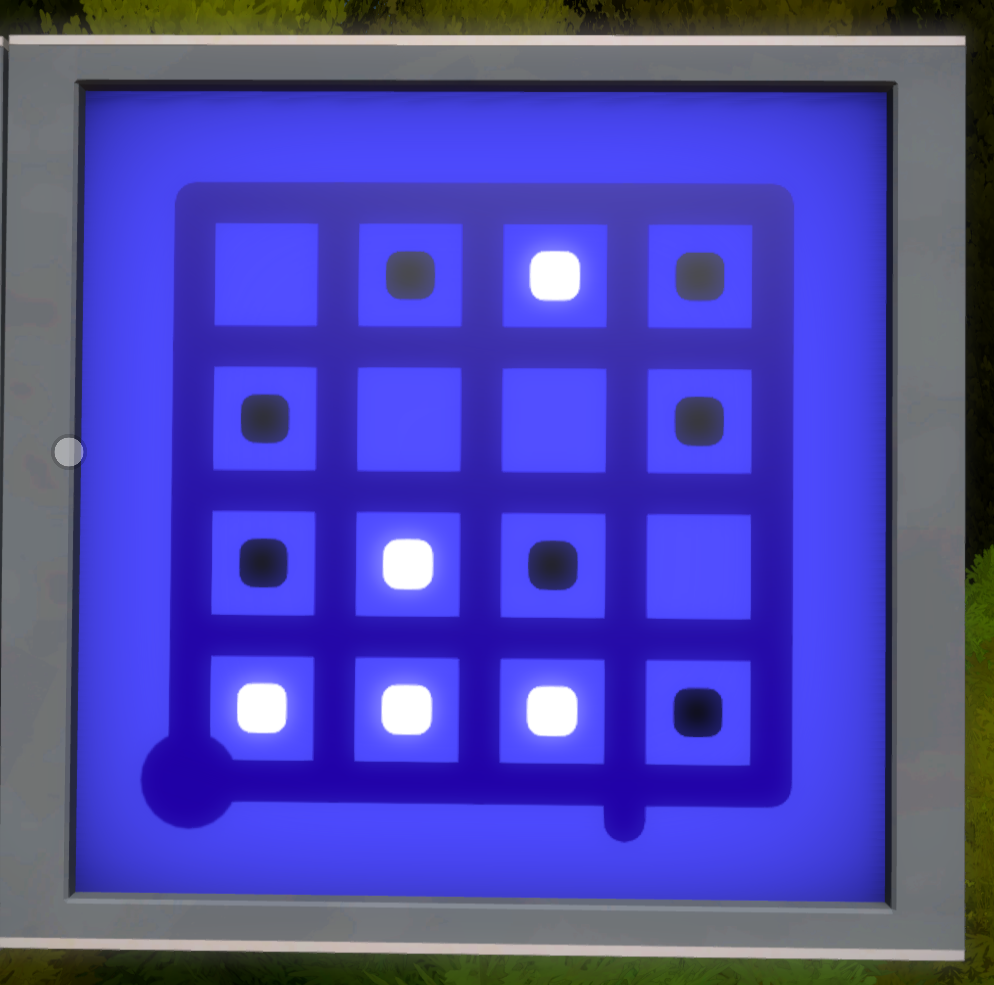## Puzzle 17

### And they throw one more loop at you by moving the end point again and removing some black squares. Start by marking all of the line segments that must be filled in and then see how to combine them. You may have to keep all of the whites together in order to solve this one. Now that these are finished turn around and head to the little hut where the green puzzles reside.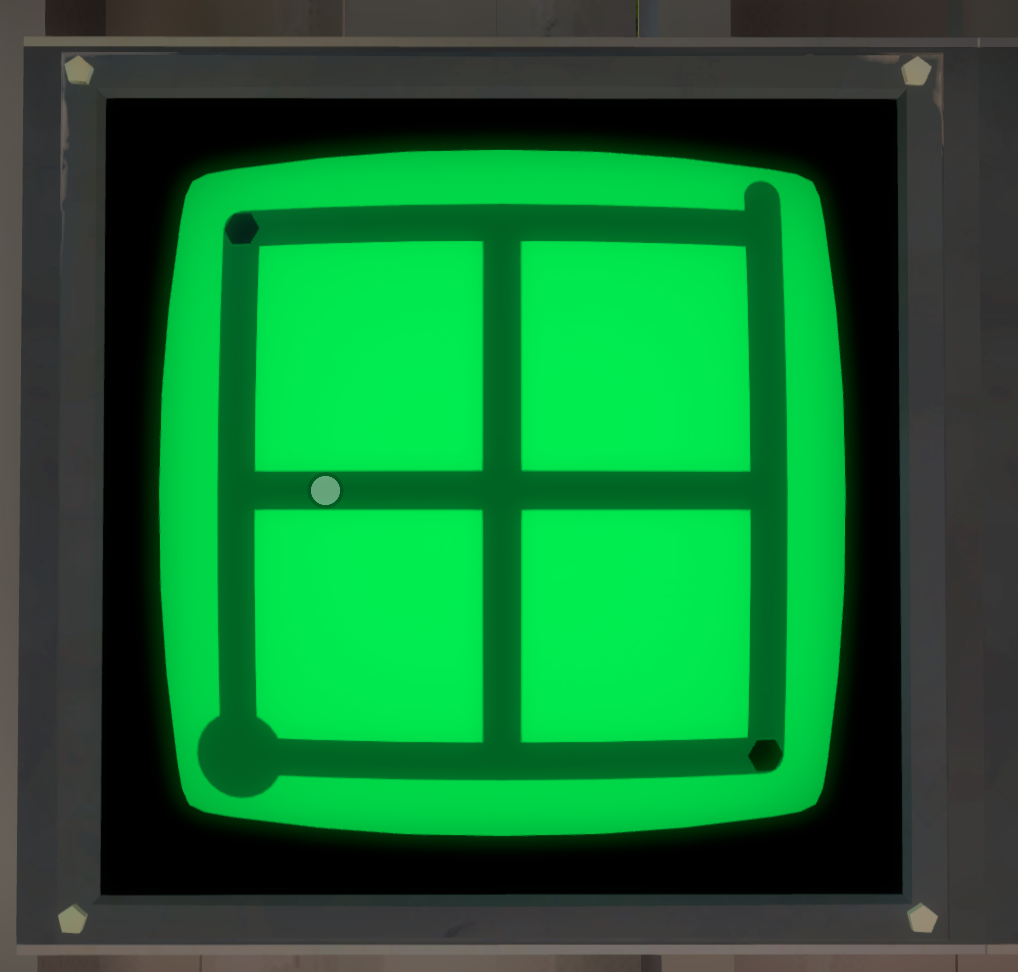## Puzzle 18

### This set of green puzzles introduces the dots on the paths. Play around and see if you can figure out the logic## Puzzle 19

### Well if you've made it here you should have realized that in order to complete these puzzles your line must pass over everyone one of the dots. If you struggle with this one try covering the dots working from top left dots first and bottom right last.## Puzzle 20

### A good trick to use on these puzzles is similar to the separation, mark the line segments that you know must be filled in. In this case it is any segment with a line in the middle or a corner with only 2 segments attached must have both segments filled in. this should really narrow down your options.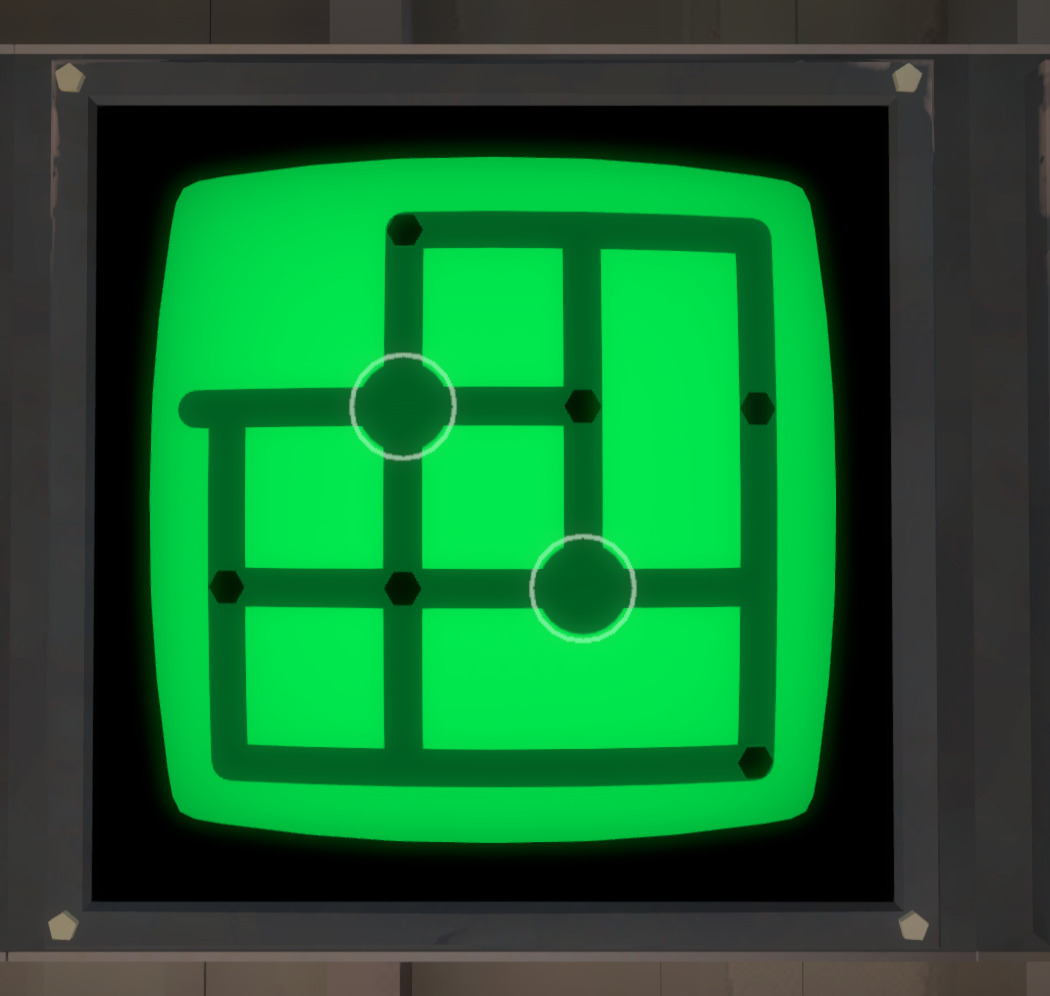## Puzzle 21

### Now you have choices on where to start. You'll have better luck starting from the bottom point and trying to grab the dots above you first and then work clockwise.## Puzzle 22

### Now there are 3 choices with where to start. See if you can eliminate any of them as a possible start based on where you must have lines. Working clockwise through the dots should help as well.## Puzzle 23

### Now that the game has taught you about black and white squares as well as dots on the lines its a good time to go back and see if you can solve the puzzle on the door you passed! You have all the knowledge necessary to tackle this! If you are having troubles maybe take a screen shot and start drawing in all the lines that you know must be there. This may help you narrow down which starting point is the one you need to use. After that its a matter of finding a way to connect your segments togethe

###### 2. Orchard - XXX Puzzles

The Starting Area is a nice introduction to a very simple concept. This concept is starting from the circle and finishing at a point that juts out of the puzzle with a rounded end.## Puzzle 1

### As you enter the orchard the game tries to introduce a new concept to you. The puzzle ahead has multiple endpoints so how do you know which is the right one? The puzzle itself doesn't seem to give you any indication so maybe there is something else around?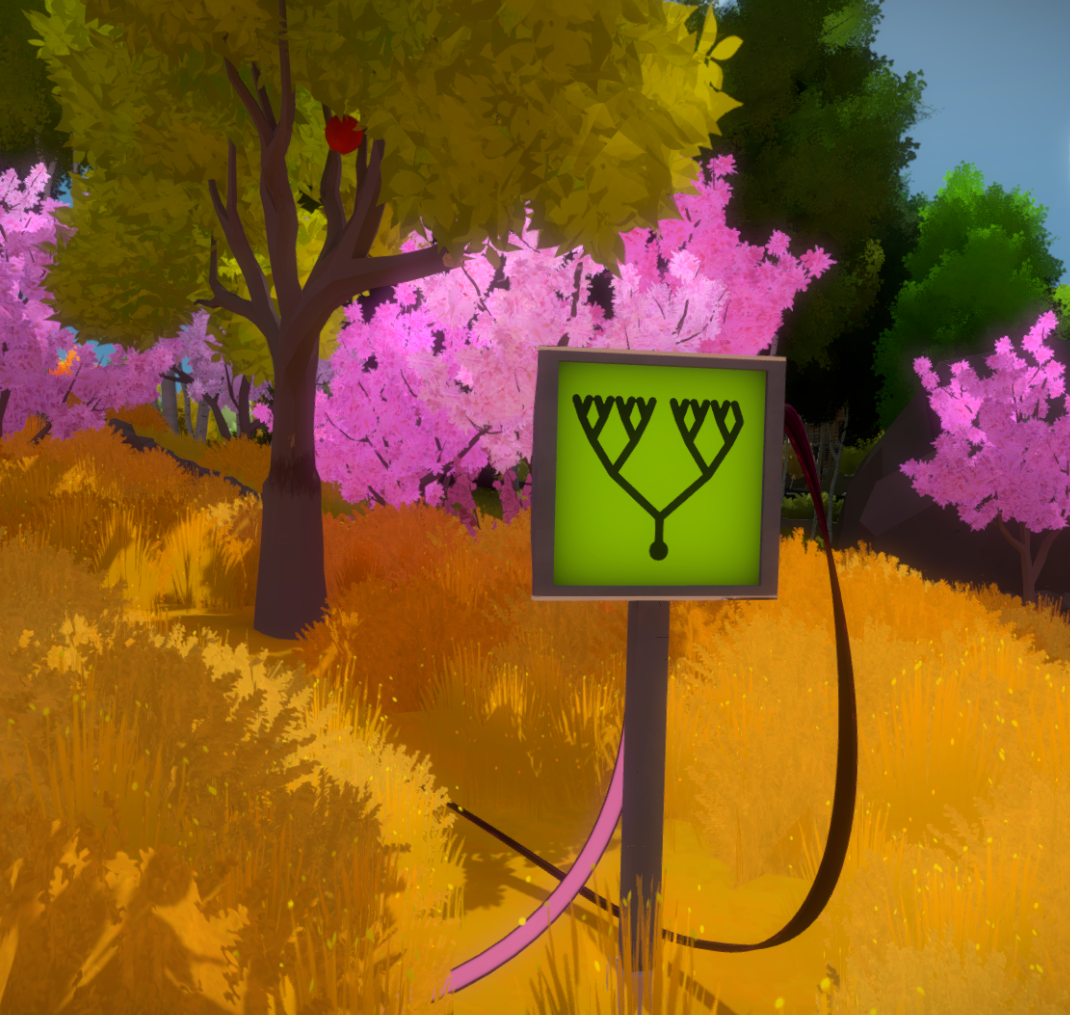## Puzzle 2

### Congrats on making it here! If you guessed your way here look out as if you get this wrong you get sent back to the previous panel! The twist they add now is a literal twist! Your clue is not as easily visible from the panel so you may have to walk and get a closer look. Note the far right endpoint that has one less fork. This will help you identify if you are looking at the clue from the proper side/direction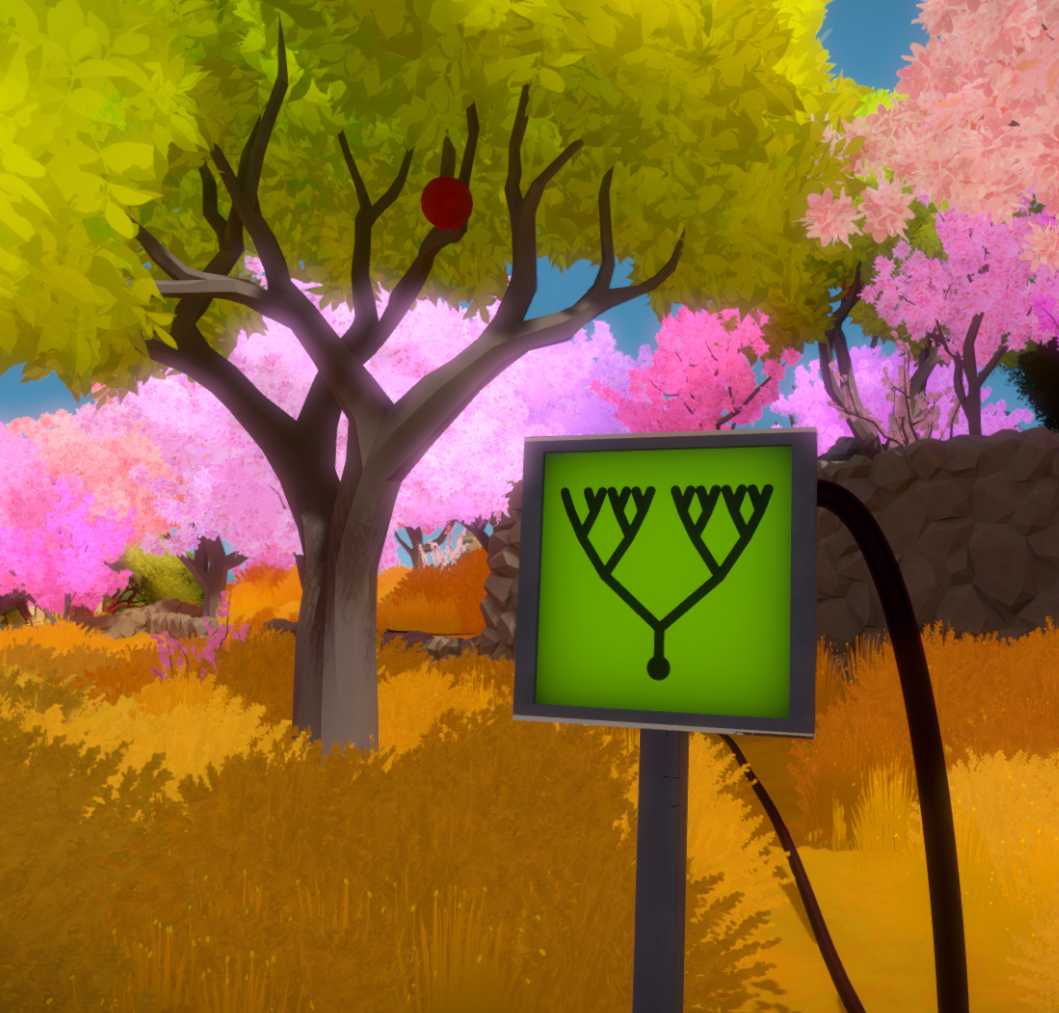## Puzzle 3

### This puzzle messes with your perspective as the branches start to cross. Again use the endpoint with less forks to help you line things up and look at it from a angle where to branches don't cross to make it easier on you to figure out.## Puzzle 4

### The twists are getting larger. again use the branches with less forks in them as your point of reference in order to figure out which end point is the correct one.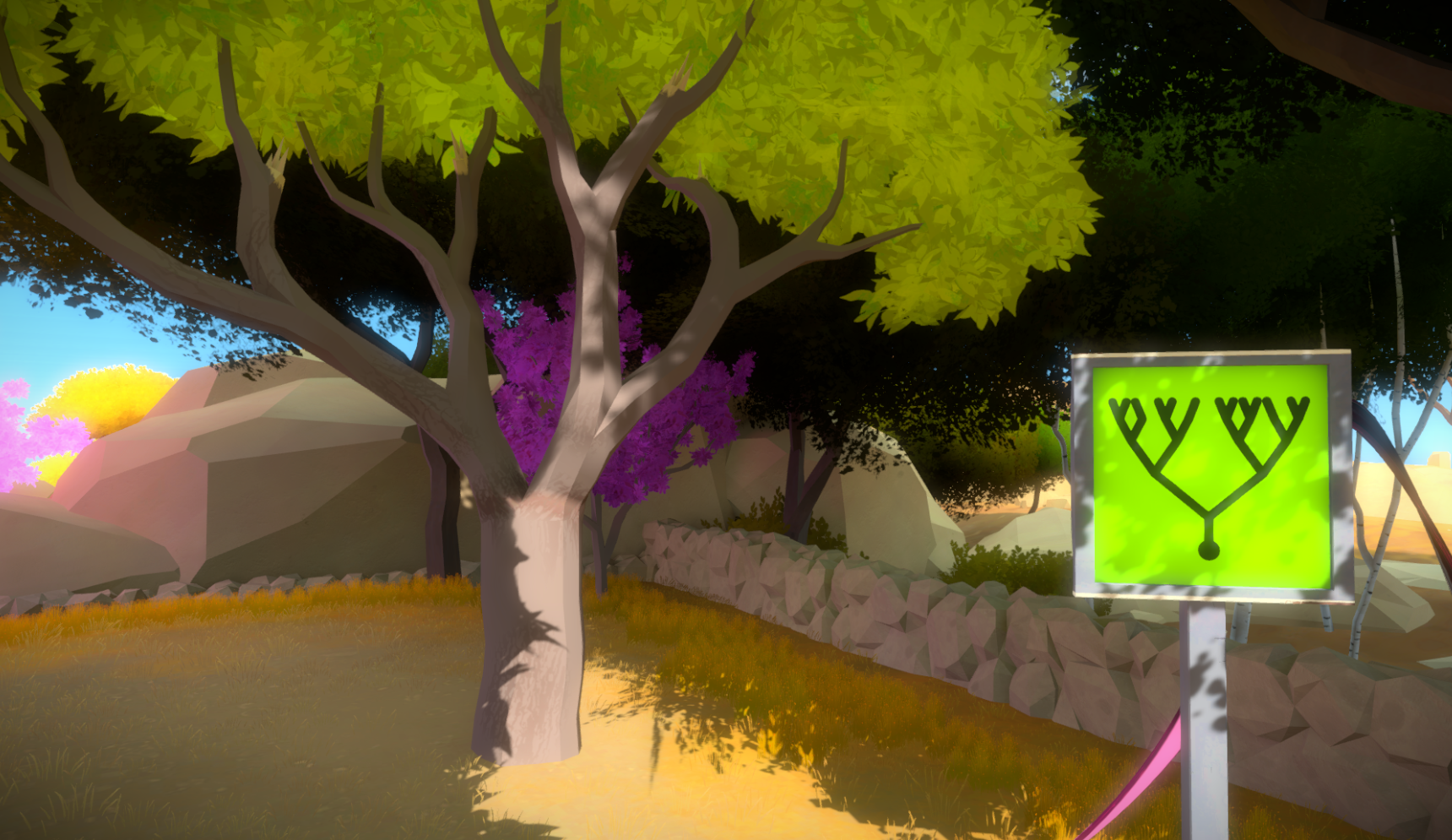## Puzzle 5

### And now the game tries to really mess with you! The obvious marker for the endpoint is missing! Whatever will you do! Take the time to compare the panel to your reference. There is a slight difference which may be an indication of where the marker used to be!

###### 3. Lake - XXX Puzzles

The Starting Area is a nice introduction to a very simple concept. This concept is starting from the circle and finishing at a point that juts out of the puzzle with a rounded end.## Puzzle 1

### The first puzzle introduces the basic concept for the game which is where to start and where to finish. Should be easy to pick up on since there is only two options for each.## Puzzle 21

### Now you have choices on where to start. You'll have better luck starting from the bottom point and trying to grab the dots above you first and then work clockwise.## Puzzle 22

### Now there are 3 choices with where to start. See if you can eliminate any of them as a possible start based on where you must have lines. Working clockwise through the dots should help as well.

###### 4. Symmetry - XXX Puzzles

The Starting Area is a nice introduction to a very simple concept. This concept is starting from the circle and finishing at a point that juts out of the puzzle with a rounded end.## Puzzle 1

### The first puzzle introduces the basic concept for the game which is where to start and where to finish. Should be easy to pick up on since there is only two options for each.## Puzzle 21

### Now you have choices on where to start. You'll have better luck starting from the bottom point and trying to grab the dots above you first and then work clockwise.## Puzzle 22

### Now there are 3 choices with where to start. See if you can eliminate any of them as a possible start based on where you must have lines. Working clockwise through the dots should help as well.

###### 5. Desert - XXX Puzzles

The Starting Area is a nice introduction to a very simple concept. This concept is starting from the circle and finishing at a point that juts out of the puzzle with a rounded end.## Puzzle 1

### The first puzzle introduces the basic concept for the game which is where to start and where to finish. Should be easy to pick up on since there is only two options for each.## Puzzle 21

### Now you have choices on where to start. You'll have better luck starting from the bottom point and trying to grab the dots above you first and then work clockwise.## Puzzle 22

### Now there are 3 choices with where to start. See if you can eliminate any of them as a possible start based on where you must have lines. Working clockwise through the dots should help as well.

###### 6. Quarry - XXX Puzzles

The Starting Area is a nice introduction to a very simple concept. This concept is starting from the circle and finishing at a point that juts out of the puzzle with a rounded end.## Puzzle 1

### The first puzzle introduces the basic concept for the game which is where to start and where to finish. Should be easy to pick up on since there is only two options for each.## Puzzle 21

### Now you have choices on where to start. You'll have better luck starting from the bottom point and trying to grab the dots above you first and then work clockwise.## Puzzle 22

### Now there are 3 choices with where to start. See if you can eliminate any of them as a possible start based on where you must have lines. Working clockwise through the dots should help as well.

###### 7. Logging/Shadows - XXX Puzzles

The Starting Area is a nice introduction to a very simple concept. This concept is starting from the circle and finishing at a point that juts out of the puzzle with a rounded end.## Puzzle 1

### The first puzzle introduces the basic concept for the game which is where to start and where to finish. Should be easy to pick up on since there is only two options for each.## Puzzle 21

### Now you have choices on where to start. You'll have better luck starting from the bottom point and trying to grab the dots above you first and then work clockwise.## Puzzle 22

### Now there are 3 choices with where to start. See if you can eliminate any of them as a possible start based on where you must have lines. Working clockwise through the dots should help as well.

###### 8. Castle/Keep - XXX Puzzles

The Starting Area is a nice introduction to a very simple concept. This concept is starting from the circle and finishing at a point that juts out of the puzzle with a rounded end.## Puzzle 1

### The first puzzle introduces the basic concept for the game which is where to start and where to finish. Should be easy to pick up on since there is only two options for each.## Puzzle 21

### Now you have choices on where to start. You'll have better luck starting from the bottom point and trying to grab the dots above you first and then work clockwise.## Puzzle 22

### Now there are 3 choices with where to start. See if you can eliminate any of them as a possible start based on where you must have lines. Working clockwise through the dots should help as well.

###### 9. Shipwreak - XXX Puzzles

The Starting Area is a nice introduction to a very simple concept. This concept is starting from the circle and finishing at a point that juts out of the puzzle with a rounded end.## Puzzle 1

### The first puzzle introduces the basic concept for the game which is where to start and where to finish. Should be easy to pick up on since there is only two options for each.## Puzzle 21

### Now you have choices on where to start. You'll have better luck starting from the bottom point and trying to grab the dots above you first and then work clockwise.## Puzzle 22

### Now there are 3 choices with where to start. See if you can eliminate any of them as a possible start based on where you must have lines. Working clockwise through the dots should help as well.

###### 10. Treehouse/Colored Pointy Circles - XXX Puzzles

The Starting Area is a nice introduction to a very simple concept. This concept is starting from the circle and finishing at a point that juts out of the puzzle with a rounded end.## Puzzle 1

### The first puzzle introduces the basic concept for the game which is where to start and where to finish. Should be easy to pick up on since there is only two options for each.## Puzzle 21

### Now you have choices on where to start. You'll have better luck starting from the bottom point and trying to grab the dots above you first and then work clockwise.## Puzzle 22

### Now there are 3 choices with where to start. See if you can eliminate any of them as a possible start based on where you must have lines. Working clockwise through the dots should help as well.

###### 11. The Bay - XXX Puzzles

The Starting Area is a nice introduction to a very simple concept. This concept is starting from the circle and finishing at a point that juts out of the puzzle with a rounded end.## Puzzle 1

### The first puzzle introduces the basic concept for the game which is where to start and where to finish. Should be easy to pick up on since there is only two options for each.## Puzzle 21

### Now you have choices on where to start. You'll have better luck starting from the bottom point and trying to grab the dots above you first and then work clockwise.## Puzzle 22

### Now there are 3 choices with where to start. See if you can eliminate any of them as a possible start based on where you must have lines. Working clockwise through the dots should help as well.

###### 12. Marsh - XXX Puzzles

The Starting Area is a nice introduction to a very simple concept. This concept is starting from the circle and finishing at a point that juts out of the puzzle with a rounded end.## Puzzle 1

### The first puzzle introduces the basic concept for the game which is where to start and where to finish. Should be easy to pick up on since there is only two options for each.## Puzzle 21

### Now you have choices on where to start. You'll have better luck starting from the bottom point and trying to grab the dots above you first and then work clockwise.## Puzzle 22

### Now there are 3 choices with where to start. See if you can eliminate any of them as a possible start based on where you must have lines. Working clockwise through the dots should help as well.

###### 13. River - XXX Puzzles

The Starting Area is a nice introduction to a very simple concept. This concept is starting from the circle and finishing at a point that juts out of the puzzle with a rounded end.## Puzzle 1

### The first puzzle introduces the basic concept for the game which is where to start and where to finish. Should be easy to pick up on since there is only two options for each.## Puzzle 21

### Now you have choices on where to start. You'll have better luck starting from the bottom point and trying to grab the dots above you first and then work clockwise.## Puzzle 22

### Now there are 3 choices with where to start. See if you can eliminate any of them as a possible start based on where you must have lines. Working clockwise through the dots should help as well.## Puzzle 1

### The first puzzle introduces the basic concept for the game which is where to start and where to finish. Should be easy to pick up on since there is only two options for each.## Puzzle 21

### Now you have choices on where to start. You'll have better luck starting from the bottom point and trying to grab the dots above you first and then work clockwise.## Puzzle 22

### Now there are 3 choices with where to start. See if you can eliminate any of them as a possible start based on where you must have lines. Working clockwise through the dots should help as well.

###### 14. Monastery - XXX Puzzles

The Starting Area is a nice introduction to a very simple concept. This concept is starting from the circle and finishing at a point that juts out of the puzzle with a rounded end.

###### 15. Peninsula - XXX Puzzles## Puzzle 1

### The first puzzle introduces the basic concept for the game which is where to start and where to finish. Should be easy to pick up on since there is only two options for each.## Puzzle 21

### Now you have choices on where to start. You'll have better luck starting from the bottom point and trying to grab the dots above you first and then work clockwise.## Puzzle 22

### Now there are 3 choices with where to start. See if you can eliminate any of them as a possible start based on where you must have lines. Working clockwise through the dots should help as well.

The Starting Area is a nice introduction to a very simple concept. This concept is starting from the circle and finishing at a point that juts out of the puzzle with a rounded end.## Puzzle 1

### The first puzzle introduces the basic concept for the game which is where to start and where to finish. Should be easy to pick up on since there is only two options for each.## Puzzle 21

### Now you have choices on where to start. You'll have better luck starting from the bottom point and trying to grab the dots above you first and then work clockwise.## Puzzle 22

### Now there are 3 choices with where to start. See if you can eliminate any of them as a possible start based on where you must have lines. Working clockwise through the dots should help as well.

###### 16. Tropical Forest - XXX Puzzles

The Starting Area is a nice introduction to a very simple concept. This concept is starting from the circle and finishing at a point that juts out of the puzzle with a rounded end.## Puzzle 1

### The first puzzle introduces the basic concept for the game which is where to start and where to finish. Should be easy to pick up on since there is only two options for each.## Puzzle 21

### Now you have choices on where to start. You'll have better luck starting from the bottom point and trying to grab the dots above you first and then work clockwise.## Puzzle 22

### Now there are 3 choices with where to start. See if you can eliminate any of them as a possible start based on where you must have lines. Working clockwise through the dots should help as well.

###### 17. Town/Hub - XXX Puzzles

The Starting Area is a nice introduction to a very simple concept. This concept is starting from the circle and finishing at a point that juts out of the puzzle with a rounded end.## Puzzle 1

### The first puzzle introduces the basic concept for the game which is where to start and where to finish. Should be easy to pick up on since there is only two options for each.## Puzzle 21

### Now you have choices on where to start. You'll have better luck starting from the bottom point and trying to grab the dots above you first and then work clockwise.## Puzzle 22

### Now there are 3 choices with where to start. See if you can eliminate any of them as a possible start based on where you must have lines. Working clockwise through the dots should help as well.

###### 18. Mountain - XXX Puzzles

The Starting Area is a nice introduction to a very simple concept. This concept is starting from the circle and finishing at a point that juts out of the puzzle with a rounded end.

###### 1. Starting/Entry Area - XXX Puzzles

The Starting Area is a nice introduction to a very simple concept. This concept is starting from the circle and finishing at a point that juts out of the puzzle with a rounded end.

###### 1. Starting/Entry Area - XXX Puzzles

The Starting Area is a nice introduction to a very simple concept. This concept is starting from the circle and finishing at a point that juts out of the puzzle with a rounded end.

This Site was created by the BJ and Co Team - 2016

This site was designed with the
.com
website builder. Create your website today.